Disclaimer: This is an example of a student written essay.

Any opinions, findings, conclusions or recommendations expressed in this material are those of the authors and do not necessarily reflect the views of UKEssays.com.

# Distance Measurement Using RSSI Method in WSN

 ✅ Paper Type: Free Essay ✅ Subject: Computer Science ✅ Wordcount: 2970 words ✅ Published: 18th Apr 2018

Distance Measurement Using RSSI Method in Wireless Sensor Networks

• Akhand Pratp Singh, Devesh Pratap Singh, Santosh Kumar

Abstract. RSSI method gives distance measurement between beacon nodes and unknown node. RSSI is Range-based localization depends on the assumption that the absolute distance between a sender and a receiver can be estimated by one or more features of the communication signal from the sender to the receiver. RSSI measurement is not more relevant because the RF signal is affected by the environment, the exact distance between the nodes cannot obtain by RSSI measurement by RSSI.

1. Introduction

Wireless Sensor Networks can be generally defined as network of nodes that cooperatively sense and control the environment enabling interaction between persons or computers and the surrounding environment. WSNs are mostly used in military surveillance, industrial process control and environmental monitoring. Node localization is a big problem of wireless sensor networks applications .According to estimation of node localization , the localization algorithms can be divided into two categories: range-based and range-free. Range-based method calculates the localization between neighboring sensors. Several ranging techniques are possible for range measurement, such as time of arrival, time difference of arrival, angle of arrival, or the receive signal strength indicator (RSSI) . Range free techniques solution depends only on the contents of received messages, which does not estimate the distance or angle between the nodes. Typical range-free localization algorithms  included Centroid, DV-Hop, Amorphous, MDS-MAP and APIT, and so on . Localization algorithm  based on range-based has higher accuracy but requires additional hardware on sensor nodes.

1. Localization of Wireless Sensor Networks

Localization  is the process by which sensor nodes determine their location. In simple terms, localization is a mechanism for discovering spatial relationships between objects. The various approaches taken in literature to solve this localization problem differ in the assumptions they make about their respective network and sensor capabilities. A detailed, but not exhaustive, list of assumptions made include assumptions about device hardware, signal propagation models, timing and energy requirements, composition of network via homogeneous vs. heterogeneous, operational environment via indoor vs. outdoor, beacon density, time synchronization, communication costs, error requirements, and node mobility . Localization of WSNs is classified in two approaches .

1. Direct Approaches

This is also known as absolute localization. The direct approach itself can be classified into two types: Manual configuration and 8GPS-based localization . The manual configuration method is very cumbersome and expensive. It is neither practical nor scalable for large scale WSNs and in particular, does not adapt well for WSNs with node mobility. The GPS-based localization method, each sensor is equipped with a GPS receiver. This method adapts well for WSNs with node mobility . However, there is a downside to this method. It is not economically feasible to equip each sensor with a GPS receiver since WSNs are deployed with 100 of 1000 of sensors. This also increases the size of each sensor, rendering them unfit for pervasive environments. Also, the GPS receivers only work well outdoors on earth and have line-of-sight requirement constraints. Such Wireless Sensor Networks can’t be used for underwater applications like habitat monitoring, water pollution level monitoring, tsunami monitoring , etc.

1. Indirect Approaches

The indirect approach  of localization is also known as relative localization  since nodes position themselves relative to other nodes in their vicinity. The indirect approaches of localization were introduced to overcome some of the drawbacks of the GPS-based direct localization techniques  while retaining some of its advantages, like accuracy of localization. In this approach, a small subset of nodes in the network, called the beacon nodes, are either equipped with GPS receivers to compute their location or are manually configured with their location. These beacon nodes then send beams of signals providing their location to all sensor nodes in their vicinity that don’t have a GPS receiver. Using the transmitted signal containing the location information, sensor nodes compute their location. This approach effectively reduces the overhead introduced by the GPS-based method. However, since the beacon nodes are also operating in the same hostile environment as the sensor nodes, they too are vulnerable to various threats, including physical capture by adversaries. This introduces new security threats concerning the honesty of the beacon nodes in providing location information Since they could have been tampered by the adversary and misbehave by providing incorrect location information. Within the indirect approach, the localization process can be classified into the following two categories.

A. Range-based

In range-based  localization, the location of a node is computed relative to other nodes in its vicinity. Range-based localization depends on the assumption that the absolute distance between a sender and a receiver can be estimated by one or more features of the communication signal from the sender to the receiver. The accuracy of such estimation, however, is subject to the transmission medium and surrounding environment. Range based techniques usually rely on complex hardware which is not feasible for WSNs since sensor nodes are highly resource-constrained and have to be produced at throwaway prices as they are deployed in large numbers. Some range-based localization techniques are as follows Angle of Arrival, Received Signal Strength Indicator (RSSI), Time of Arrival and Time Difference of Arrival. In this paper we are discussing about the RSSI technique , RSSI technique does need require additional hardware, which will not increase the hardware cost and the size of the nodes. However, due to RF signals influenced by the environment, the exact distance between the nodes cannot obtain by using RSSI , so the localization accuracy of nodes are not high.

B. Range-free

Range-free localization never tries to estimate the absolute point to point distance based on received signal strength or other features of the received communication signal like time, angle, etc. This greatly simplifies the design of hardware, making range-free methods very appealing and a cost-effective alternative for localization in WSNs. Typical range-free localization algorithms included Centroid ,DV-Hop, Amorphous, MDS-MAP and APIT,etc.

RSSI measurement  calculates the signal loss in the dissemination process with the theory or experience loss of signal propagation model and distance calculated between transceiver to receiver by path distance formulae. Some measure terms which are important role in RSSI measurement as follows:

1. Path Loss Model

Path loss models  are free space propagation model, the logarithmic distance path loss model, Hata model, etc. the logarithmic distance path loss model  is shown by formula (1):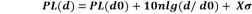(1)

Where d is distance from transmitter to receiver and its unit is km, n is path loss exponent that measures the rate at which the RSSI decreases with distance and the value of n depends on the specific propagation environment, Xσ is a zero mean Gaussian distributed random variable whose mean value is 0 and it reflects the change of the received signal power in certain distance, d0 is reference distance and usually equals 1 meter, PL(d0) is a known reference power value in dBmilliwatts at a reference distance d0 from the transmitter.

1. Received Signal Power at Reference distance

Suppose A is the received signal power in the distance d0 between trans- mitter and receiver, the formula (2) can be generated.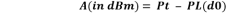(2)

Where Pt is power of transmitter and PL(d0) is a known reference power value in dBmilliwatts at a reference distance d0 from the transmitter.

1. Distance Calculated by RSSI measurement

The RSSI Value at the certain distance is calculated by the given formula.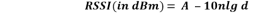(3)

Where RSSI is the received signal power. A is the received signal power in the sdistance of 1meter,n is the path loss index and relates to the environment. Then we select maximum RSSI value and then we convert it into distance by given formulae. After calculating the RSSI values we can obtain the maximum value of the RSSI which is known as RSSImax.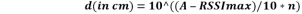(4)

Where RSSImax is the maximum received signal power selected from all the RSSI values. A is the received signal power in the distance of 1meter,n is the path loss index and relates to the environment.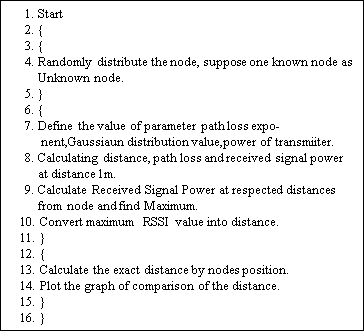When we go through the RSSI method then we have to go through the following step of the algorithms as follows:

1. Result and Analysis

Our simulation is done in 10m x 10m two dimensional environment. Node deployment accuracy is very important. 9 nodes are deployed randomly we can get their coordinate and suppose one known node as unknown node and then we can find the distances, path loss, Gaussian distributed value .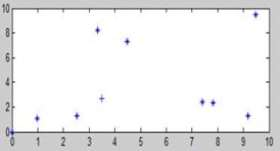Figure 1 Random deployed node

Where +: unknown node

*: Beacon node

In the simulation we assume (x1,y1): (3.4855, 2.7068) as unknown Node and further we calculate the distance, maximum RSSI value in Scenario of 9 node where one node suppose to be mobile by RSSI Method when n=2 ,A=8.4734 dBm and power loss at reference distance is 31.5266 dBm.

Table1.Distance Calculation

 Sensor Position (X,Y) Distance from(3.4855,2.7068) Distance Power vaue(dBm) RSSI Vaue(dBm) Max.RSSI vaue(dBm) Converted Distance(m) (9.1800,1.2861) 5.8689 66.9458 -26.9201 (9.1800,1.2861) 9.0471 75.6011 -35.5755 (3.3232,8.1869) 5.4825 65.5835 -25.5578 (2.5261,1.2651) 1.7318 42.5354 -2.5098 -2.5098 1.5726 (4.4869,7.3112) 4.7120 62.5547 -22.5291 (7.4019 ,2.3911) 3.9291 58.9203 -18.8947 (0.9715,1.1163) 2.9749 53.3566 -13.3310 (7.8191,2.3079) 4.3519 60.9643 -20.9387

When we simulate we found that distance measure by RSSI principle is 1.5726 meter, but when we applied the distance formulae for the Coordinate we found that exact distance is 5.4825.So we found that there is measure margin of error.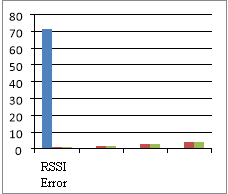Figure2. Error in distance calculated by RSSI

In figure1 we can see that the distance calculated by RSSI is not accurate, because the error percentage is 71.35.

1. Conclusions

Localization performance will depend on many things, including the localization algorithm used, the quantity of prior coordinate information, the method selected, and the accuracies possible from those measurements in the environment of interest. The RSSI measurement is studied in this paper, but this method is not more accurate because the radio frequency signals is affected by the environment, the exact distance between the nodes cannot obtain by RSSI measurement. Experimental measurement and simulation results show that the distance is obtain, but measurement is not accurate. The proposed method is a good option in wireless sensor node localization, because of low cost and less complexity of the simulation. In future we can work on improving the RSSI method for the more accuracy because sometimes there is problem of accurate distance and it depends only on the measurement parameter model. The result shows that in future if we work through the RSSI method for the specific scenarios like war (soldier) and forest fire then the method may provide the specific result and maybe there is need of some more Improvement in this proposed method because some time the result shown by experiment is out of bound so there is need of some more improvement.

References

 Yick J., Mukherjee B. and Ghosal D., “Wireless Sensor Network survey”, Elsevier Computer Network, vol.52, pp. 2292– 2330, 2008.

 Mao G., BarÄ±s F. and Anderson B.D.O.,“Wireless Sensor Network Localization Techniques”, Elsevier Computer Networks, vol.51,pp. 2529–2553, 2007.

 Zheng J., Wu C., Chu H. and Xu Y., “An Improved RSSI Measurement In Wireless Sensor Networks”, Elsevier Procedia Engineering, vol.15, pp. 876 – 880, 2011.

 Patwari N., Aah J. N. and Kyperountas S., “Locating the nodes cooperative localization In Wireless Sensor Network”, IEEE Signal Processing Magazine, vol.22, No.4, pp. 54-69, 2005.

 Srinivasan A. and Wu J., “A Survey on Secure Localization in Wireless Sensor Networks”, Florida Atlantic University, USA.

 Amundson I. and Koutsoukos X.D., “A Survey on Localization for Mobile Wireless Sensor Networks”,Computer Science,Springer,vol.5801, pp. 235-254, 2009.

 Han G., Xu H.,Duong R.Q., Jiang J.,and Hara T., “Localization algorithms of Wireless Sensor Networks: a survey”, Telecommunication Systems, Springer, vol. 52, Issue 4, pp. 2419-2436, 2013.

 Zeng Y., Cao J., Hong J, Zhang S. And Xie L., “Secure localization and location very fication in wireless sensor networks: a survey”, Springer, vol. 64, Issue 3, pp. 685-701, 2013.

 Wei X. and Qi Y., “Research on Some Key Techniques of Wireless Sensor Network”, Electrical Engineering, Springer, vol. 127, pp. 255-260, 2012.

 Heo J, Kim J. H. and Hong C.S., “A Logical Group Formation and Management Mechanism Using RSSI for Wireless Sensor Networks”, Computer Science,  Springer, vol. 5297, pp. 207-216, 2008 .

 Erdogan S.Z., “Mobility Monitoring by Using RSSI in Wireless Sensor Net Works”,Computer and Information Science, Springer, vol. 90, pp. 572-580, 2010.

 Adewumi O., Djouani K.,and Kurien A., “Performance Evaluation of RSSI Based Distance Measurement for Localization in Wireless Sensor Networks”, Social Informatics and Telecommunications Engineering, Springer, vol.119, pp. 74-83, 2013.

 Ahn H., Lee Y.H., Cho H.J., Rhee S.B., and Lee J.H., “A RSSI-Based Approach for Localization of Wireless Sensor Network in Indoor”, Electrical  Engineering, Springer, vol. 120, pp. 123-127, 2012.

 Miao C., Dai G., Mao K., Li Y., and Chen Q., “RI-MDS: Multidimensional Scaling Iterative Localization Algorithm Using RSSI in Wireless Sensor Networks”, Computer and Information Science, Springer, vol. 501, pp. 164- 175, 2015.

 Shen X., Wang Z., Jiang P., Lin R., and Sun Y., “Connectivity and RSSI Based Localization Scheme for Wireless Sensor Networks”, Computer Sci- ence, Springer, vol. 3645, pp. 578-587, 2005.

View all

## DMCA / Removal Request

If you are the original writer of this essay and no longer wish to have your work published on UKEssays.com then please:

Related Services

Prices from

\$USD152

Approximate costs for:

• 1000 words
• 7 day deliveryHumanity University

Dedicated to your worth and value as a human being!

Related Lectures